# Partially Erasing Entanglement: Measuring a GHZ Triplet into a Bell Pair

02 Sep 2015

Recently, while trying grok delayed choice quantum erasers by simplifying/adjusting/toying-around-with the problem, I stumbled onto an improvement for my solution to the quantum network flow puzzle I posted months ago.

In this post: how to "erase" a qubit out of a GHZ state.

# GHZ Triplets and Bell Pairs

A Bell pair is a set of two qubits in a superposition of all-OFF and all-ON, i.e. in the state $\frac{1}{\sqrt{2}} \ket{00} + \frac{1}{\sqrt{2}} \ket{11}$. A GHZ state is like a Bell pair, but with more qubits involved. For example, a GHZ triplet is a set of three qubits in the state $\frac{1}{\sqrt{2}} \ket{000} + \frac{1}{\sqrt{2}} \ket{111}$.

You might expect that the qubits in a GHZ state are "more entangled" than the qubits in a Bell pair, since the superposition is larger, but actually the reverse is true. Because of the monogamy of entanglement, qubits in a Bell pair are more entangled with each other than qubits in a GHZ state are. The third qubit in a GHZ triplet tends to be more of a third wheel than a useful resource, as far as doing-fun-things-with-quantum goes.

Because Bell pairs can be used for some tasks that GHZ states can't do (e.g. superdense coding), it's useful to be able to reduce a GHZ state into a Bell pair by kicking one of the qubits out. Previously, I thought that the only way to do this was to hit the unwanted qubit with a controlled-not controlled by one of the other involved qubits. This clears the unwanted qubit by toggling its value in the all-ON part of the superposition while leaving it alone in the all-OFF part of the superposition.

The controlled-not approach works fine, but it requires the unwanted qubit to be in the same place as one of the other qubits (because of the quantum controlled operation). Satisfying that condition will, usually, require moving qubits around (i.e. you need some quantum channels with available bandwidth).

But it turns out that it's possible to avoid paying that quantum bandwidth cost. By hitting the qubit with a Hadamard gate to obscure its value, measuring it, and using the measurement outcome to fix a phase parity issue, you only need to use classical bandwidth. (I call this "erasing" the qubit only because of how I stumbled onto the idea, not because of the actual operations that are happening. I don't know the proper existing name for the technique.)

# Circuit Manipulation

I think that the easiest way to understand why the "erasure" approach works, and how, is to start from the circuit for the controlled-not approach and apply several simple obviously-correct transformations.

We'll start with a circuit that creates a GHZ triplet, then uses a controlled-not to kick the third qubit out of the GHZ state: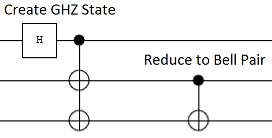After the third qubit has been cleared, we can hit it with whatever operations we want (because it's not being used for anything anymore). Using the power of informed foresight, we'll hit it with a Hadamard gate and then a measurement: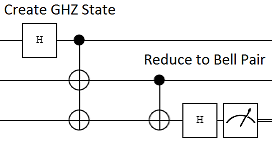Now it's time to hop the Hadamard gate over the Not gate. This is allowed, but it transforms the value-toggling Not gate into a phase-toggling Z gate (because $H \cdot X = Z \cdot H$):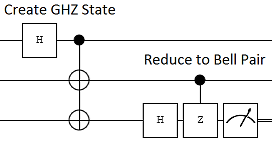Z gates are a bit like controlled operations, in that they have no effect on qubits that are OFF. As a result, exchanging a Z gate with one of its controls doesn't change its effect. Let's do it: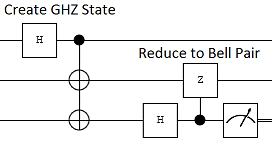Having the control on the third wire is useful because controls commute with measurements (i.e. classical conditions are equivalent to quantum conditions). This allows us to perform the phase correction after the measurement instead of before: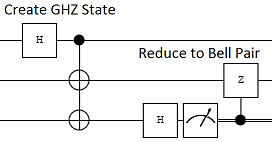That's it, we're done! The final circuit:

1. Starts in the state $\ket{000}$.
2. Creates a GHZ triplet state $\frac{1}{\sqrt{2}} \ket{000} + \frac{1}{\sqrt{2}} \ket{111}$.
3. Hits the third qubit with a Hadamard, transitioning to the state $\frac{1}{2} \ket{000} + \frac{1}{2} \ket{001} + \frac{1}{2} \ket{110} - \frac{1}{2} \ket{111}$.
4. Measures the third qubit, collapsing the system into either the state $\frac{1}{\sqrt{2}} \parens{\ket{00} + \ket{11}} \ket{0}$ or the state $\frac{1}{\sqrt{2}} \parens{\ket{00} - \ket{11}} \ket{1}$.
5. Fixes the minus sign in the third-qubit-was-ON outcome, with a Z gate controlled by the measurement outcome.
6. Finishes with the first two qubits unconditionally in the Bell pair state $\frac{1}{\sqrt{2}} \ket{00} + \frac{1}{\sqrt{2}} \ket{11}$.

We still had to send information about the third qubit to the second qubit, but now the transmitted information is classical (i.e. a measurement result) instead of quantum (i.e. the original qubit). This technique also works for larger GHZ states involving more qubits: you can eject qubits from the state one by one by Hadamarding+measuring+conditional-Z-a-qubit-still-in-the-state-ing them.

# Updated Puzzle Solution

Because my solution to the previously posted quantum network flow puzzle involves kicking a qubit out of a GHZ state, using "erasure" to do the kicking allows a couple parts of the network to be downgraded from quantum to classical.

Here is a data flow diagram of the improved solution: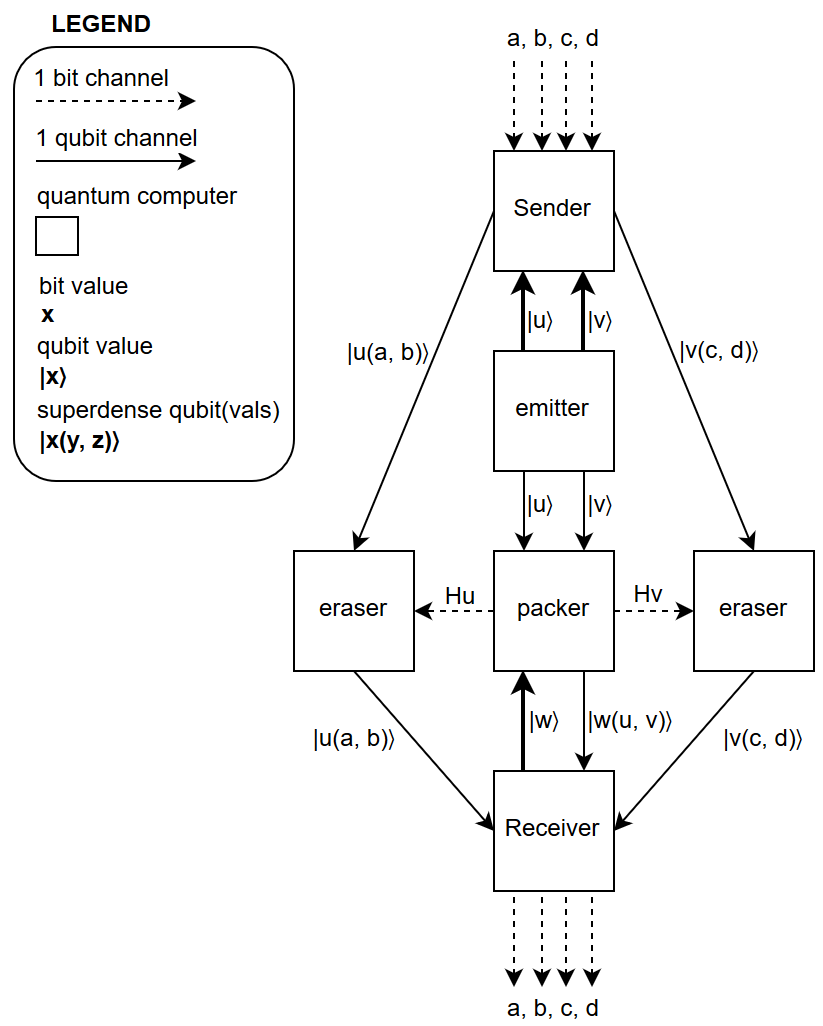I won't bore you with the worked out details of the solution. It's obvious what changes to make, given the content of the puzzle post and of this post.

# Summary

A qubit can be kicked out of a GHZ state by measuring it along a spin axis perpendicular to the entanglement axis, and using the measurement result to perform a phase correction.